Courses

# Substitution method of Integration, Business Mathematics & Statistics B Com Notes | EduRev

## B Com : Substitution method of Integration, Business Mathematics & Statistics B Com Notes | EduRev

The document Substitution method of Integration, Business Mathematics & Statistics B Com Notes | EduRev is a part of the B Com Course Business Mathematics and Statistics.
All you need of B Com at this link: B Com

## Integration by Substitution

"Integration by Substitution" (also called "u-substitution") is a method to find an integral, but only when it can be set up in a special way.

The first and most vital step is to be able to write our integral in this form: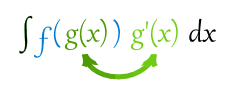Note that we have g(x) and its derivative g'(x)

Like in this example: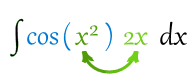Here f=cos, and we have g=x2 and its derivative of 2x
This integral is good to go!

When our integral is set up like that, we can do this substitution: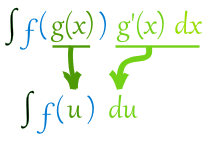Then we can integrate f(u), and finish by putting g(x) back as u.

Like this:

### Example: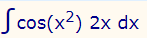We know (from above) that it is in the right form to do the substitution: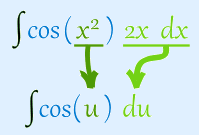Now integrate: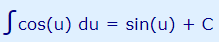|
And finally put u=x2 back again:
sin(x2) + C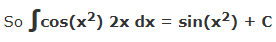worked out really nicely! (Well, I knew it would.)

This method only works on some integrals of course, and it may need rearranging:
Example: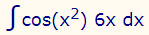Oh no! It is 6x, not 2x. Our perfect setup is gone.

Never fear! Just rearrange the integral like this: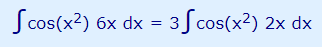(We can pull constant multipliers outside the integration, see Rules of Integration.)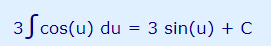Now put u=x2 back again:
3 sin(x2) + C
Done!
Now we are ready for a slightly harder example:
Example: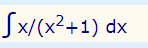Let me see ... the derivative of x2+1 is 2x ... so how about we rearrange it like this: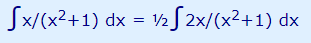Then we have: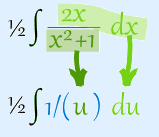Then integrate: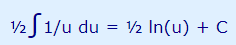Now put u=x2+1 back again:
½ ln(x2+1) + C
Example: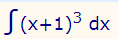Let me see ... the derivative of x+1 is ... well it is simply 1.

So we can have this: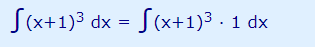Then we have: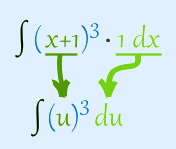Then integrate: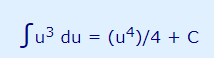Now put u=x+1 back again:## In Summary

• When we can put an integral in this form: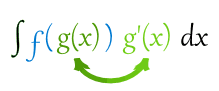• Then we can make u=g(x) and integrate ∫f(u) du
• And finish up by re-inserting g(x) where u is.
Offer running on EduRev: Apply code STAYHOME200 to get INR 200 off on our premium plan EduRev Infinity!

122 videos|142 docs

,

,

,

,

,

,

,

,

,

,

,

,

,

,

,

,

,

,

,

,

,

,

,

,

;